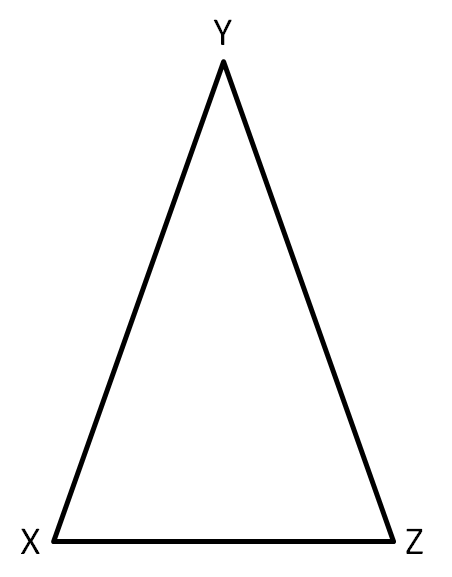# Line of Reflections on Isosceles Triangles

Directions: How many ways can you determine the location of the line of reflection for isosceles triangle XYZ that maps Point X to Point Z?### Hint

– What tools can you use to figure this out?
– Can you use a compass to figure this out in more than one way?
– How can you measure to find it?

We know of five ways:
– Use a compass to find the perpendicular bisector
– Use a compass to find the angle bisector
– Measure the midpoint by finding half of the length of the base by using a ruler
– Cover up the triangle by moving left to right along the base until the vertex between the congruent sides is touched.
– Trace the triangle with patty paper and fold it in half to form two congruent right triangles. Draw the line along the fold.

Source: Irvine Math Project, Nanette Johnson, and Robert Kaplinsky.

## Similar Triangles 2

Directions: Using the digits 0 to 9 at most one time each, create two similar …

1.The only answer I can think of is the perpendicular bisector of segment XZ. Is the task to find this in more than one way? (compass and straight edge, or ruler to measure half and protractor to measure 90 degrees). If I am missing something, please post the answer in the answer section of this page.

•Robert Kaplinsky

Hi Ben. Somehow the problem got copied into the hint and the hints got copied into the answer. I fixed it and listed the five ways we’ve figured out. Let me know what you think.

2.How about finding where the angle bisectors of X and Z cross, then joint that point to the vertex Y.

Also, draw the perpendicular from X to segment ZY and from z to segment XY, then connect that point to Y.

Both of these work too.

3.Find any point of concurrency (incenter, circumcenter, orthocenter or centroid)and connect that point with the vertice of the vertex angle to form the perpendicular bisector of the base.